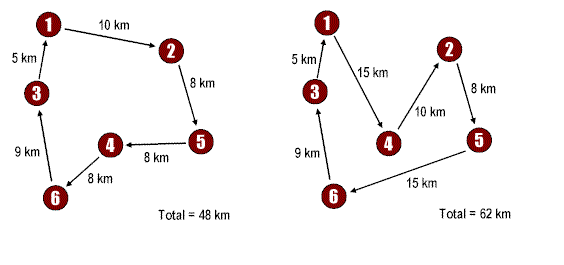The travelling salesman problem is a classical mathematical problem solved through graph theory, actually the problem was – How can a Travelling salesman starting from one city can return to same city while travelling various city at Shortest route while  travelling?According to the graph theory, this requirement makes a closed graph or it can be termed as Hamilton Cycle ( A cycle is tour in graph where initial vertex is repeated at end) and this particular graph is called Hamilton Graph.Above figures represent two routes starting from one and returning back at 1 , it is clearly it is selection of route that makes complete short tour.

The Travelling Salesman Problem (also known as TSP) is a algorithmic problem in the field of computer science.

“Solving Travelling Salesman Problem through Dynamic Programming”

The  rule for writing the  Dynamic Programming is as follows-

F(Sn, DN) = RN+ FN-1*(Sn-1, Dn-1)

Where-

F (SN, DN ) = Return on n-stage of the value input

SN= Initial Conditions

SN-1 = Final Condition

DN= Decision made at each stage.

DN-1= Decision made at final stage

RN= Return function

FN= Final function

Implementation

• Determine the basis of Hamilton graph using-

f(i, ∅)=∁i,1;2≤i≤n)

• Calculate f(i,s) using

F(i,s)=min{cij+f(j,s-{j})

• By obtained results of previous step calculate the equation of a recursive relationship

f(1,v-1)=min⁡(ci,k+f(K,V-{1,K})

• After calculating above step the recursive relationship will give shortest rule.

EXAMPLE-Implementation in C++

#include<stdio.h>

#include<conio.h>

#include<iostream>

using namespace std;

int c = 0,cost = 999;

int graph = { {0, 20, 25, 30},

{20, 0, 45, 35},

{25, 45, 0, 40},

{30, 35, 40, 0}

};

void swap (int *a, int *b)

{

int temp;

temp = *a;

*a = *b;

*b = temp;

}

void copy_array(int *x, int n)

{

int i, sum = 0;

for(i = 0; i <= n; i++)

{

sum += graph[x[i % 4]][x[(i + 1) % 4]];

}

if (cost > sum)

{

cost = sum;

}

}

void permute(int *x, int i, int n)

{

int j, k;

if (i == n)

{

copy_array(a, n);

}

else

{

for (j = i; j <= n; j++)

{

swap((x + i), (x + j));

permute(x, i + 1, n);

swap((x + i), (x + j));

}

}

}

int main()

{

int i, j;

int a[] = {0, 1, 2, 3};

permute(a, 0, 3);

cout<<“minimum cost:”<<cost<<endl;

getch();

}

Importance of Travelling Salesman Problem

The Travelling Salesman problem is best for calculating optimal route. It is used in many practical solutions. Not only minimum route the shortest timing can also be calculated. It is used in logistic planning and goods allocation. Still it has its importance in research too.

Author:
Category :
Publish Date :
Social Sharing